# Practice Quiz in Field Effect Transistor Amplifiers Part 3

(Last Updated On: February 3, 2020)

This is the Online Practice Quiz in Field Effect Transistor Amplifiers Part 3 from the book, Electronic Devices and Circuit Theory 10th Edition by Robert L. Boylestad. If you are looking for a reviewer in Electronics Engineering this will definitely help. I can assure you that this will be a great help in reviewing the book in preparation for your Board Exam. Make sure to familiarize each and every questions to increase the chance of passing the ECE Board Exam.

### Continue Part III of the Online Practice Quiz

#### Quiz in Field Effect Transistor Amplifiers

Question 21. The E-MOSFET is quite popular in ________ applications.

A. digital circuitry

B. high-frequency

C. buffering

D. All of these

Question 22. Referring to this figure, calculate the value of RD if the ac gain is 10. Assume VGSQ = ¼Vp.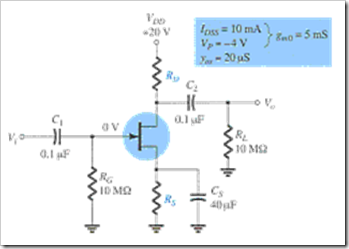A. 2.2 kΩ

B. 2.42 kΩ

C. 2.62 kΩ

D. 2.82 kΩ

Question 23. Referring to this figure, calculate Av if rd = 19 kΩ.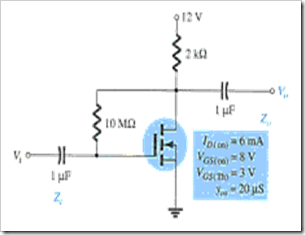A. –2.85

B. –3.26

C. –2.95

D. –3.21

Question 24. When VGS = 0.5 Vp gm is ________ the maximum value.

A. one-fourth

B. one-half

C. three-fourths

D. None of these

Question 25. Which of the following is a required condition to simplify the equations for Zo and Av for the self-bias configuration?

A. rd ≤ 10RD

B. rd = RD

C. rd ≥ 10RD

D. None of these

Question 26. The steeper the slope of the ID versus VGS curve, the ________ the level of gm.

A. less

B. same

C. greater

D. None of these

Question 27. Referring to this figure, calculate Av for yos = 58 µS.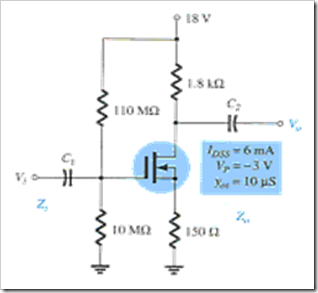A. –7.29

B. –7.50

C. –8.05

D. –8.55

Question 28. Determine the value for RD if the ac gain is 8.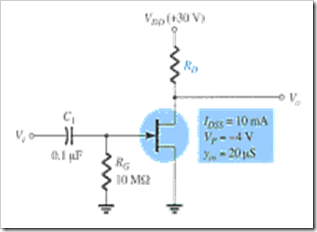A. 1.51 kΩ

B. 1.65 kΩ

C. 1.85 kΩ

D. 2.08 kΩ

Question 29. Referring to this figure, find Zo if yos = 20 µS.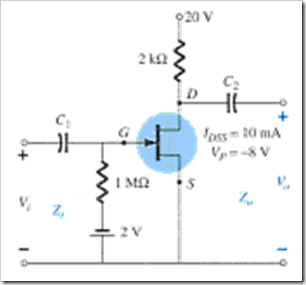A. 1.85 kΩ

B. 1.92 kΩ

C. 2.05 kΩ

D. 2.15 kΩ

Question 30. Referring to the transfer characteristics shown below, calculate gm at VGSQ = –1 V.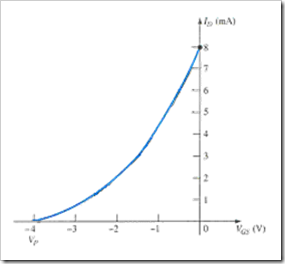A. 2 mS

B. 3 mS

C. 4 mS

D. 5 mS

### More Practice Quiz in Field Effect Transistor Amplifiers

Practice Quiz Part 1

Practice Quiz Part 2

Practice Quiz Part 3

Practice Quiz Part 4

Practice Quiz Part 5

Practice Quiz Part 6

### See: Complete List of Practice Quizzes

Note: After taking this particular quiz, you can proceed to check all the topics.

Please do Subscribe on YouTube!

P inoyBIX educates thousands of reviewers and students a day in preparation for their board examinations. Also provides professionals with materials for their lectures and practice exams. Help me go forward with the same spirit.

“Will you subscribe today via YOUTUBE?”

Subscribe